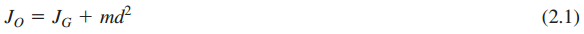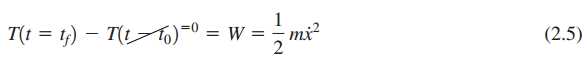### Create an Account

Home / Questions / Examine Eqs 2. and 2.5 and verify that the units dimensions of the different terms in the ...

# Examine Eqs 2. and 2.5 and verify that the units dimensions of the different terms in the respective equations are consistent A vibratory system has a mass

1. Examine Eqs. (2.1) and (2.5) and verify that the units (dimensions) of the different terms in the respective equations are consistent.2. A vibratory system has a mass m = 10 kg, k = 1500 N/m, and c = 2500 N/(m/s). Given that the displacement response has the form x(t) = 0.2sin(9t) m, plot the graphs of , the spring force kx, and the damper force versus time and discuss them.

May 16 2020 View more View LessSubscribe To Get Solution